### Home > CALC > Chapter 8 > Lesson 8.1.5 > Problem8-51

8-51.
1.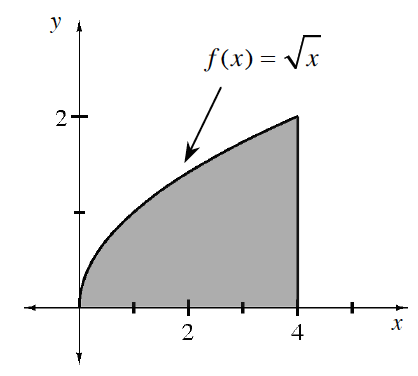Using the methods you have learned, find the volume of the region bounded by: y =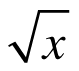, y = 0, and x = 4,
revolved about the lines below. Use the steps outlined in problem 8-49. Homework Help ✎

1. x-axis

2. y-axis

3. x = −2

4. y = 5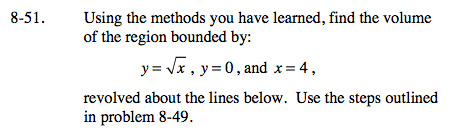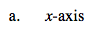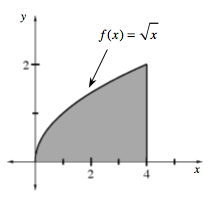After sketching an arbitrary rectangle (with Δx→0), it is apparent that there will not NOT be a hole in the center of this solid.
That indicates that the Disk Method will be appropriate.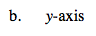This arbitrary rectangle indicates that there will be a hole in the center of this solid. You will have to use the Washer Method.

Horizontal rectangles indicate that the bounds and the integrand will be in terms of y.

Notice that the figure will be a cylinder with a curvy hole in the center.
The radii of the cylinder is R = 4.
The radii of the curvy hole is r = y².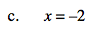Q: Washers or disks? Think: Will there be a hole or won't there?

Q: Will the integral be in terms of x or y? Think: Are the rectangles vertical or horizontal?

Notice that these radii are 2 units longer than the radii in part (b).
R = 2+4
r = 2 + y²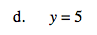R = 5

$\text{Since }y=\sqrt{x}\text{ represents the distance between the }x\text{-axis and }y=\sqrt{x},\text{ it follows that: }r=5-\sqrt{x}$